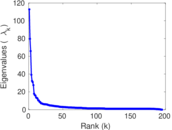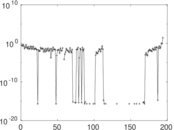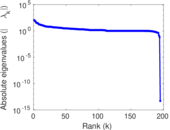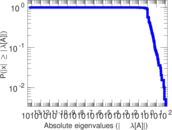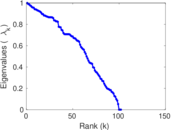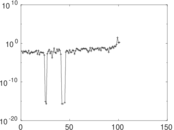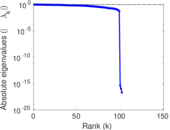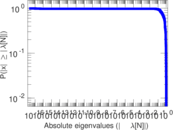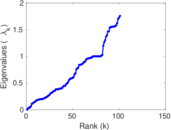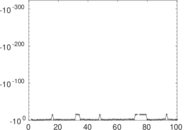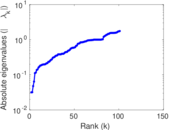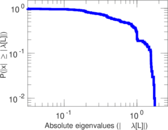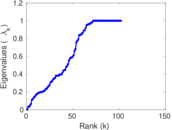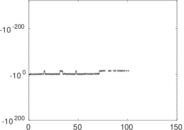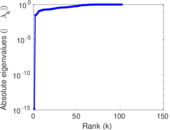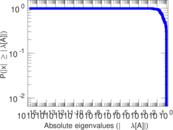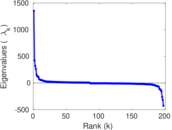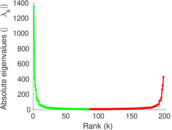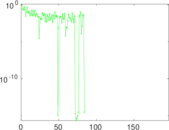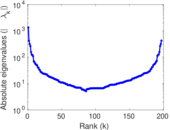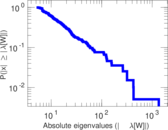# Wiktionary edits (dv)

This is the bipartite edit network of the Divehi Wiktionary. It contains users and pages from the Divehi Wiktionary, connected by edit events. Each edge represents an edit. The dataset includes the timestamp of each edit.

 Code `mdv` Internal name `edit-dvwiktionary` Name Wiktionary edits (dv) Data source http://dumps.wikimedia.org/ AvailabilityDataset is available for download Consistency checkDataset passed all tests Category Authorship network Dataset timestamp 2017-10-20 Node meaning User, article Edge meaning Edit Network formatBipartite, undirected Edge typeUnweighted, multiple edges Temporal dataEdges are annotated with timestamps

## Statistics

 Size n = 1,361 Left size n1 = 197 Right size n2 = 1,164 Volume m = 4,497 Unique edge count m̿ = 2,131 Wedge count s = 121,051 Claw count z = 7,451,735 Cross count x = 423,415,319 Square count q = 66,886 4-Tour count T4 = 1,024,014 Maximum degree dmax = 1,168 Maximum left degree d1max = 1,168 Maximum right degree d2max = 129 Average degree d = 6.608 38 Average left degree d1 = 22.827 4 Average right degree d2 = 3.863 40 Fill p = 0.009 293 18 Average edge multiplicity m̃ = 2.110 28 Size of LCC N = 967 Diameter δ = 12 50-Percentile effective diameter δ0.5 = 3.531 95 90-Percentile effective diameter δ0.9 = 5.509 69 Median distance δM = 4 Mean distance δm = 4.059 81 Gini coefficient G = 0.763 130 Balanced inequality ratio P = 0.190 794 Left balanced inequality ratio P1 = 0.120 970 Right balanced inequality ratio P2 = 0.247 054 Relative edge distribution entropy Her = 0.819 505 Power law exponent γ = 2.871 93 Tail power law exponent γt = 2.041 00 Tail power law exponent with p γ3 = 2.041 00 p-value p = 0.000 00 Left tail power law exponent with p γ3,1 = 1.711 00 Left p-value p1 = 0.149 000 Right tail power law exponent with p γ3,2 = 2.151 00 Right p-value p2 = 0.000 00 Degree assortativity ρ = −0.044 171 5 Degree assortativity p-value pρ = 0.041 462 5 Spectral norm α = 112.778 Algebraic connectivity a = 0.031 638 1 Spectral separation |λ1[A] / λ2[A]| = 1.416 47 Controllability C = 826 Relative controllability Cr = 0.680 395

## Plots

### Fruchterman–Reingold graph drawing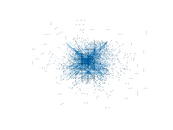### Degree distribution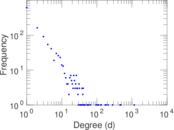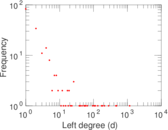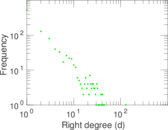### Cumulative degree distribution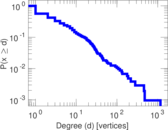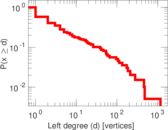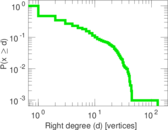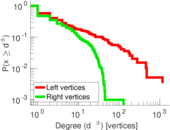### Lorenz curve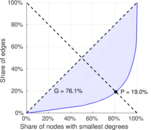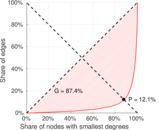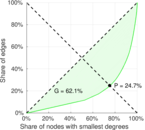### Spectral distribution of the adjacency matrix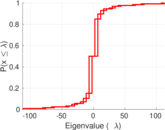### Spectral distribution of the normalized adjacency matrix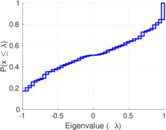### Spectral distribution of the Laplacian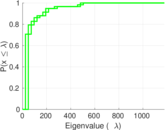### Spectral graph drawing based on the adjacency matrix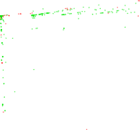### Spectral graph drawing based on the Laplacian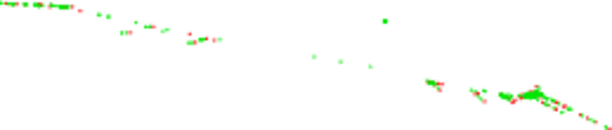### Spectral graph drawing based on the normalized adjacency matrix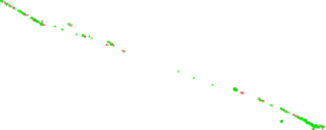### Degree assortativity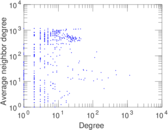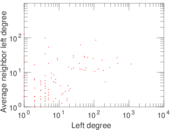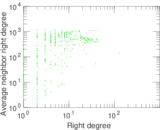### Zipf plot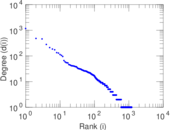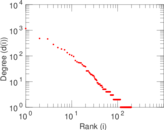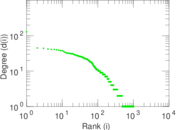### Hop distribution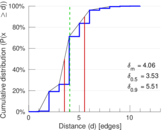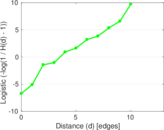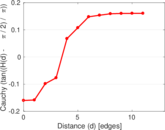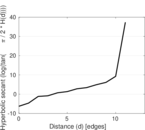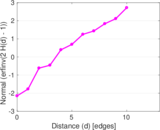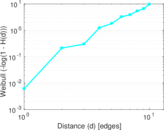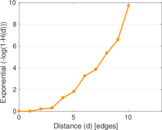### Double Laplacian graph drawing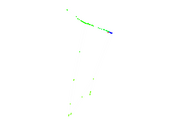### Delaunay graph drawing### Edge weight/multiplicity distribution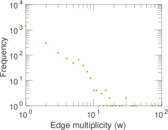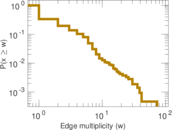### Temporal distribution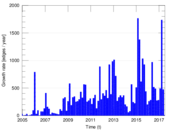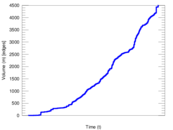### Temporal hop distribution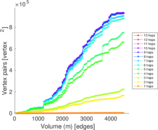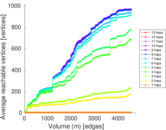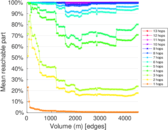### Diameter/density evolution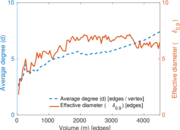### Matrix decompositions plots